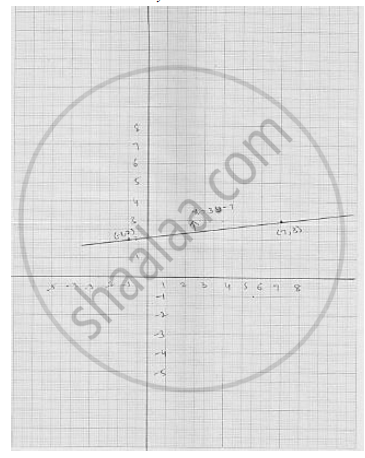# Draw the Graph of the Following Linear Equation in Two Variable : (X-2)/3 = Y - 3 - Mathematics

Draw the graph of the following linear equation in two variable :  (x-2)/3 = y - 3

#### Solution

We have

(x-2)/3 = y - 3

⇒  x - 2 = 3  (y -3)

⇒  x - 2 = 3y - 9

⇒  x = 3y - 9 + 2

⇒ x = 3y - 7

Putting  y = 0 , we get   x - 0  = -7 ⇒ x = - 7

Putting  y = 2 , we get  x - 3 (2) = - 7 ⇒ x =-1

Putting  y = 3  , we get  x = 3  ( 3)  - 7 ⇒ x = 2

Thus, we get the following table giving the two points on the line represented by the
equation  (x -2)/y  = y - 3

Graph for the equation   (x -2)/y  = y - 3Concept: Graph of a Linear Equation in Two Variables
Is there an error in this question or solution?

#### APPEARS IN

RD Sharma Mathematics for Class 9
Chapter 7 Linear Equations in Two Variables
Exercise 7.3 | Q 1.7

Share